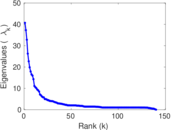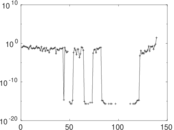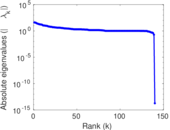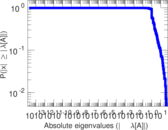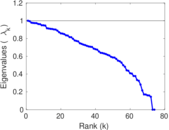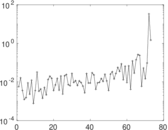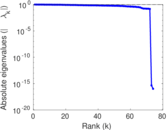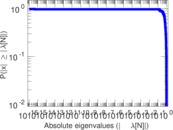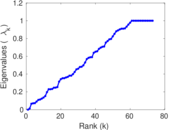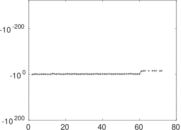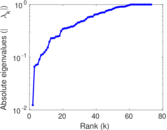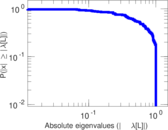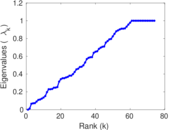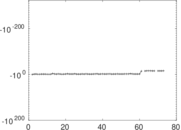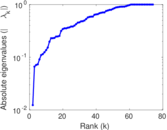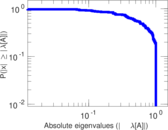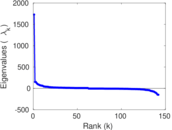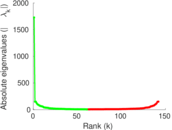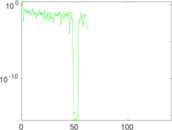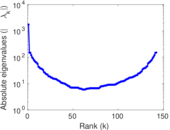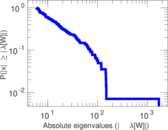# Wikinews edits (th)

This is the bipartite edit network of the Thai Wikinews. It contains users and pages from the Thai Wikinews, connected by edit events. Each edge represents an edit. The dataset includes the timestamp of each edit.

 Code `nth` Internal name `edit-thwikinews` Name Wikinews edits (th) Data source http://dumps.wikimedia.org/ AvailabilityDataset is available for download Consistency checkDataset passed all tests Category Authorship network Dataset timestamp 2017-10-20 Node meaning User, article Edge meaning Edit Network formatBipartite, undirected Edge typeUnweighted, multiple edges Temporal dataEdges are annotated with timestamps

## Statistics

 Size n = 1,737 Left size n1 = 142 Right size n2 = 1,595 Volume m = 2,960 Unique edge count m̿ = 2,212 Wedge count s = 344,269 Claw count z = 66,066,050 Cross count x = 10,831,037,346 Square count q = 18,966 4-Tour count T4 = 1,536,468 Maximum degree dmax = 797 Maximum left degree d1max = 797 Maximum right degree d2max = 46 Average degree d = 3.408 18 Average left degree d1 = 20.845 1 Average right degree d2 = 1.855 80 Fill p = 0.009 766 44 Average edge multiplicity m̃ = 1.338 16 Size of LCC N = 1,544 Diameter δ = 13 50-Percentile effective diameter δ0.5 = 3.373 79 90-Percentile effective diameter δ0.9 = 5.603 22 Median distance δM = 4 Mean distance δm = 3.899 74 Gini coefficient G = 0.680 217 Balanced inequality ratio P = 0.228 209 Left balanced inequality ratio P1 = 0.126 351 Right balanced inequality ratio P2 = 0.346 284 Relative edge distribution entropy Her = 0.768 082 Power law exponent γ = 4.660 09 Tail power law exponent γt = 2.541 00 Tail power law exponent with p γ3 = 2.541 00 p-value p = 0.000 00 Left tail power law exponent with p γ3,1 = 1.691 00 Left p-value p1 = 0.873 000 Right tail power law exponent with p γ3,2 = 2.781 00 Right p-value p2 = 0.000 00 Degree assortativity ρ = −0.354 200 Degree assortativity p-value pρ = 2.222 30 × 10−66 Spectral norm α = 40.689 0 Algebraic connectivity a = 0.012 355 6 Spectral separation |λ1[A] / λ2[A]| = 1.091 76 Controllability C = 1,440 Relative controllability Cr = 0.837 209

## Plots

### Fruchterman–Reingold graph drawing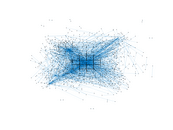### Degree distribution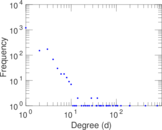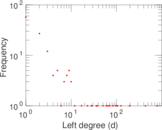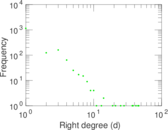### Cumulative degree distribution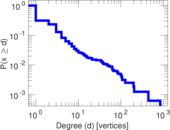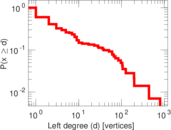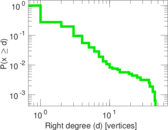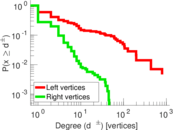### Lorenz curve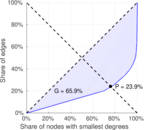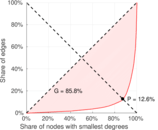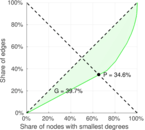### Spectral distribution of the adjacency matrix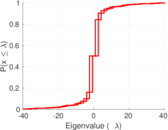### Spectral distribution of the normalized adjacency matrix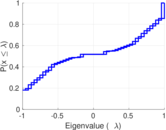### Spectral distribution of the Laplacian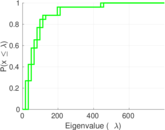### Spectral graph drawing based on the adjacency matrix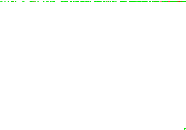### Spectral graph drawing based on the Laplacian### Spectral graph drawing based on the normalized adjacency matrix### Degree assortativity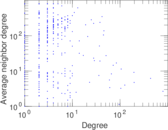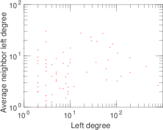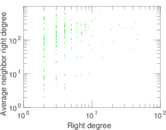### Zipf plot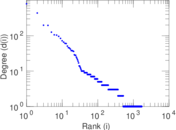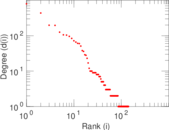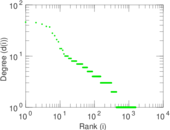### Hop distribution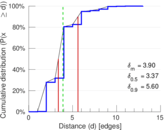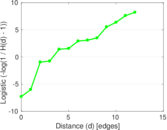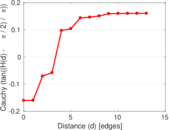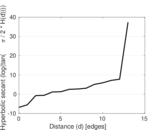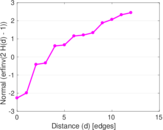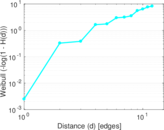### Double Laplacian graph drawing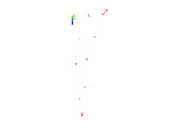### Delaunay graph drawing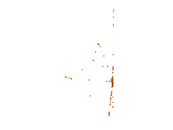### Edge weight/multiplicity distribution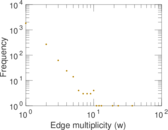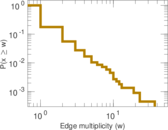### Temporal distribution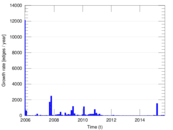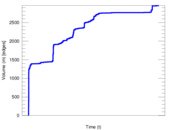### Temporal hop distribution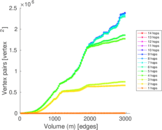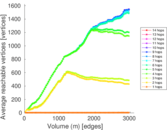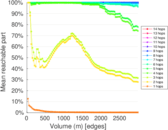### Diameter/density evolution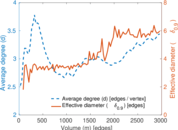### Matrix decompositions plots